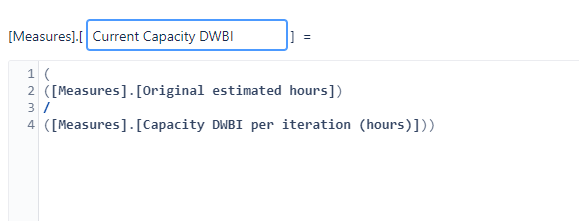# Original Estimated hours for specific projects Calculated Measure

Hello,
I have several projects selected on my table and i have a calculated measure like this one for every project selected althought is there any way to add/change this formula so that the original estimated hours is from a certain project and not all of the projects selected.In other words, for this formula above is there a way to get the original estimated hours only for the DWBI project. Im not the best with calculated measures. Thanks in Advance!

Hello Goncalo_Marques,
Welcome to the eazyBI community!

You need to define a tuple to get a measure value for one specific dimension member.
For example, this calculation will show the original estimated hours of the project with the name ‘DWBI’.

``````([Measures].[Original estimated hours]
,[Project].[DWBI])
``````

Using DefaultMember and CurrentHierarchyMember is often useful in tuples. For example, the next formula will show the share of DWBI project as % of All projects (DefaultMember).

``````([Measures].[Original estimated hours]
,[Project].[DWBI])
/
([Measures].[Original estimated hours]
,[Project].DefaultMember)
``````

Also, you could consider putting the Project dimension in rows or in columns (or pages) - the initial generic formula [Measures].[Original estimated hours] would show the hours for each project by default. This, however, would require that the denominator formula is also generic and not specific to one project.

I hope this helps.

Best,
Ilze

1 Like